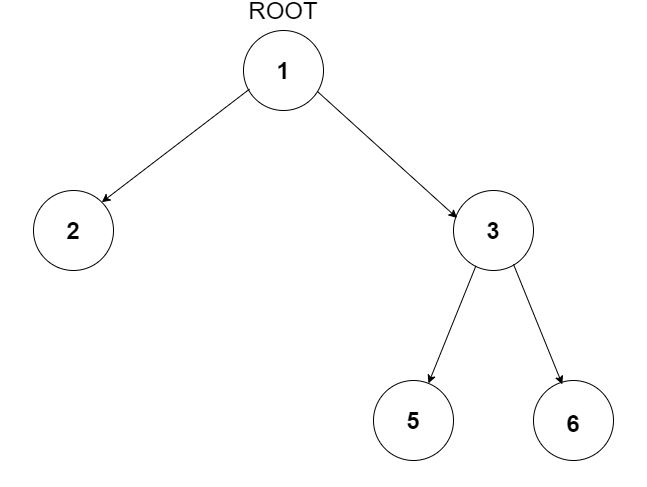# Triplets in Binary Tree

Posted: 27 Aug, 2020
Difficulty: Easy

## PROBLEM STATEMENT

#### You have been given a Binary Tree of integers and an integer 'X'. Find all the triplets in the tree whose sum is strictly greater than 'X'. The nodes in the triplet must hold the relationship of grandparent-parent-child.

##### For example:
``````For the given binary tree and X = 9
````````````{1, 3, 6} is a valid triplet because 6 is a node whose parent is 3 and grand-parent is 1. Also, the sum of these nodes is 1 + 3 + 6 = 10 which is strictly greater than X = 9.
``````
##### Input Format:
``````The first line contains an integer 'T' which denotes the number of test cases or queries to be run. Then the test cases follow.

The first line of each test case contains a single integer 'X'.

The second line of each test case contains elements in the level order form. The line consists of values of nodes separated by a single space. In case a node is null, we take -1 on its place.

For example, the input for the tree depicted in the below image will be:
````````````1
2 3
4 -1 5 6
-1 7 -1 -1 -1 -1
-1 -1
``````

#### Explanation :

``````Level 1 :
The root node of the tree is 1

Level 2 :
Left child of 1 = 2
Right child of 1 = 3

Level 3 :
Left child of 2 = 4
Right child of 2 = null (-1)
Left child of 3 = 5
Right child of 3 = 6

Level 4 :
Left child of 4 = null (-1)
Right child of 4 = 7
Left child of 5 = null (-1)
Right child of 5 = null (-1)
Left child of 6 = null (-1)
Right child of 6 = null (-1)

Level 5 :
Left child of 7 = null (-1)
Right child of 7 = null (-1)

The first not-null node(of the previous level) is treated as the parent of the first two nodes of the current level. The second not-null node (of the previous level) is treated as the parent node for the next two nodes of the current level and so on.
The input ends when all nodes at the last level are null(-1).
``````
##### Note :
``````The above format was just to provide clarity on how the input is formed for a given tree.

The sequence will be put together in a single line separated by a single space. Hence, for the above-depicted tree, the input will be given as:

1 2 3 4 -1 5 6 -1 7 -1 -1 -1 -1 -1 -1
``````
##### Output Format:
``````For each test case, print a single line containing three space-separated integers denoting all the triplets.

Triplets must be in (grand-parent, parent, child) fashion, each separated by a single space.

The output of each test case will be printed in a separate line.
``````
##### Note:
``````1. Return the triplets in any order but each triplet must follow (grand-parent, parent, child) fashion.

2. You are not required to print the expected output, it has already been taken care of. Just implement the given function.
``````
##### Constraints:
``````1 <= T <= 10 ^ 2
0 <= X <= 10 ^ 9
0 <= N <= 10 ^ 3
-10^9 <= NODE.DATA <= 10^9

Where 'N' is the total number of nodes in the binary tree, and 'NODE.DATA' is the value of a node.

Time Limit: 1 sec.
``````Approach 1

We will try to keep track of the grand-parent, parent, and child (i.e. the current node) while traversing in the tree. For this, we will have the main three parameters in the recursion function, one for each type.

• recursion(Node* gParent, Node* parent, Node* child ....)

While calling the children nodes of the current node

• Parent of the current node becomes GrandParent of children nodes
• The current node becomes Parent for children nodes

If at any node, the sum of grandParent, parent, and child becomes more than X, the given integer, we will push this triplet to a global array ‘Triplets’ which will hold all such triplets.